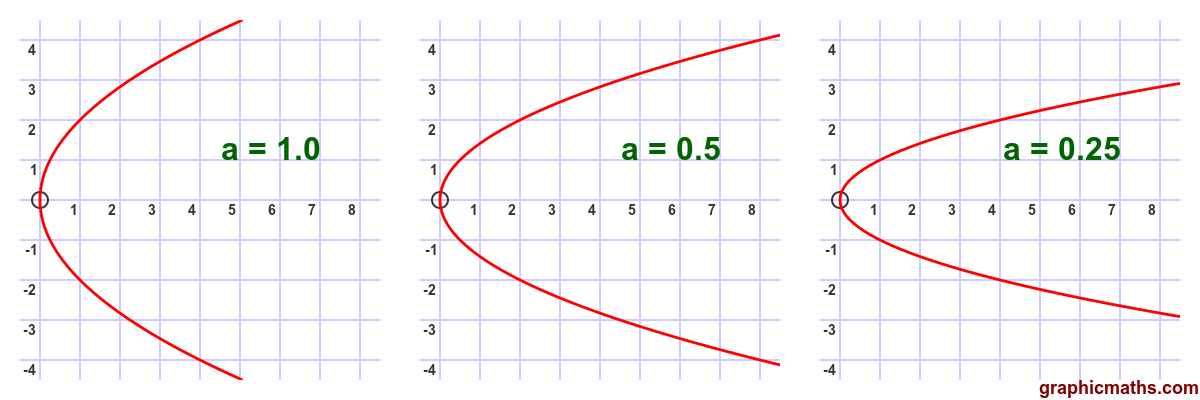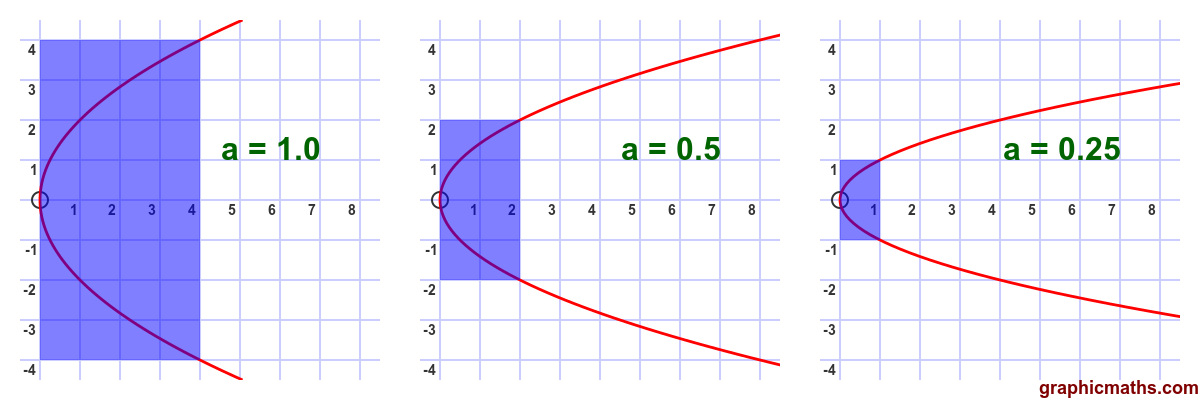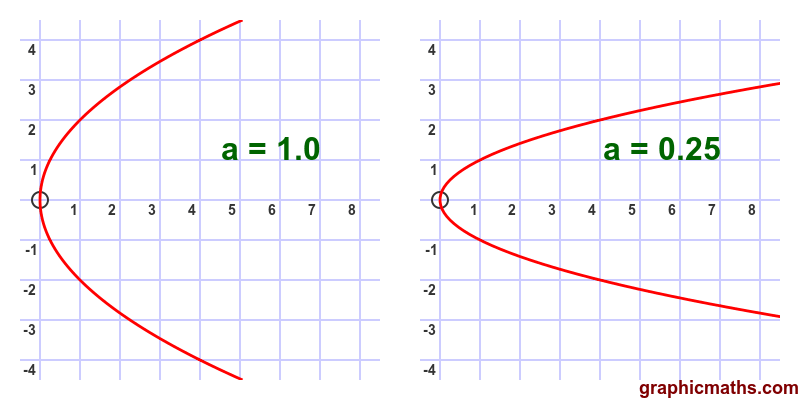# Parabolas - effect of parameter 'a'

By Martin McBride, 2020-09-13
Tags: parabola cartesian equation
Categories: coordinate systems pure mathematicsThis section looks at the effect of changing the parameter $a$ in the Cartesian equation of the parabola $y^2 = 4 a x$.

Here is the same parabola, plotted with different values of $a$:Changing the value of $a$ moves the position of the focus and the directrix, which in turn changes the curve. The smaller the value of $a$, the closer the focus and directrix are to the origin.

## All parabolas are the same shape

An interesting fact about parabolas is that they are all similar (ie the same shape). Making $a$ smaller makes the entire parabola smaller, but doesn't change its shape. Other curves have the same property, for example all circles are the same shape - changing the radius makes a different sized circle, but doesn't change its shape.

This shouldn't really be a surprise. When we make $a$ smaller, we move the focus and directrix closer together, but we then draw the parabola in exactly the same way, so of course we just end up with a smaller parabola of the exact same shape.

But that may not be entirely obvious from looking at the image above - as $a$ get smaller the parabola appears to be narrower and more pointed. That is simply because you can see more of the shape. In this diagram, the blue shaded rectangle shows the equivalent portion of the curve for each value of $a$. If you look closely you will see that they are identical shapes:As a further illustration, in this animation we draw two parabolas, with $a = 1$ and $a = 0.25$, and zoom in on the smaller parabola until they are both the same size: